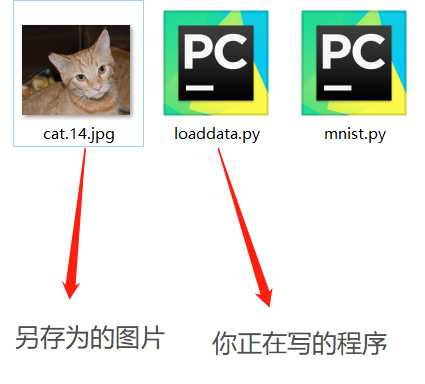# Pytorch读取，加载图像数据(一)

• Github

• 关注公众号：tuduisuinian（土堆碎念），菜单底部可以获取pytorch教程PDF文档

## 零：准备## 壹：数据集的准备## 贰：用其他库读取图像文件

• 使用`matplotlib`库进行图像的读取

`matplotlib`中的函数跟Matlab很像。

`imread（文件地址）`：进行读取图像的操作（参数为读取图像文件的路径）

`imshow（数组）`：进行图像的显示操作（显示图像的数组）

`show（）`：显示一个窗口，用于显示图像（很多时候，不显示图像的话，是忘记使用这个函数）

``````import matplotlib.pyplot as plt

#图片的高H为460，宽W为346，颜色通道C为3
print(img.shape)
print(img.dtype)
print(type(img))
plt.imshow(img)
plt.show()``````

``````(460, 346, 3)
uint8
<class ‘numpy.ndarray‘>``````

``````import matplotlib.pyplot as plt

img = [img1, img2]
for i in img:
plt.imshow(i)
plt.show()``````

• 使用`cv2`进行图像的读取

`cv2`库中，需要用到的函数有：

`imread（文件地址）`：读取地址处的文件图像

`imshow(‘窗口名称‘, 图像数组)`：将图像数组显示出来，但必须结合`waitKey()`使用，否则无法显示图像

`waitKey(延迟时间)`：需要设置延迟时间，当延迟时间≤0时，窗口将会一直延迟，延迟无穷长时间，按下任一按键，可以继续执行下面程序。当延迟时间＞0，即窗口图像会显示对应毫秒后，自动消失。

``````import cv2
print(img1.shape)
print(img1.dtype)
print(type(img1))
cv2.imshow(‘img‘, img1)
cv2.waitKey(0)``````

``````(460, 346, 3)
uint8
<class ‘numpy.ndarray‘>``````

Matplotlib与cv2对图像的数据格式的处理是相似的，但是也是有区别的：

• 使用`PIL`库进行图像处理

`PIL`全称为`Python Image Library`。是给Python提供图像处理相关的库。

`open（文件地址）`：打开文件，注意是打开，并没有读取。主要作用是保持检查文件地址，同时保证文件是打开的状态。当对图像处理的时候，会自动加载。

`show()`：使用系统自带的图像查看器，查看图像

``````from PIL import Image
img1 = Image.open(‘002.jpg‘)
# 因为PIL有自己的数据结构，所以没有shape,dtype属性
# print(img1.shape)
# print(img1.dtype)
print(type(img1))
img1.show()``````

``<class ‘PIL.JpegImagePlugin.JpegImageFile‘>``

``````import matplotlib.pyplot as plt
from PIL import Image
import numpy as np

img1 = Image.open(‘002.jpg‘)
img1 = np.array(img1)
print(img1.shape)
print(img1.dtype)
plt.imshow(img1)
plt.show()``````

``````(460, 346, 3)
uint8``````

## 叁：总结

• `matplotlib`中的`imread`,`imshow`,`show`函数
• `cv2`中的`imread`,`imshow`,`waitKey`函数
• `PIL`中的`open`,`show`函数

Pytorch读取，加载图像数据(一)

(0)
(0)